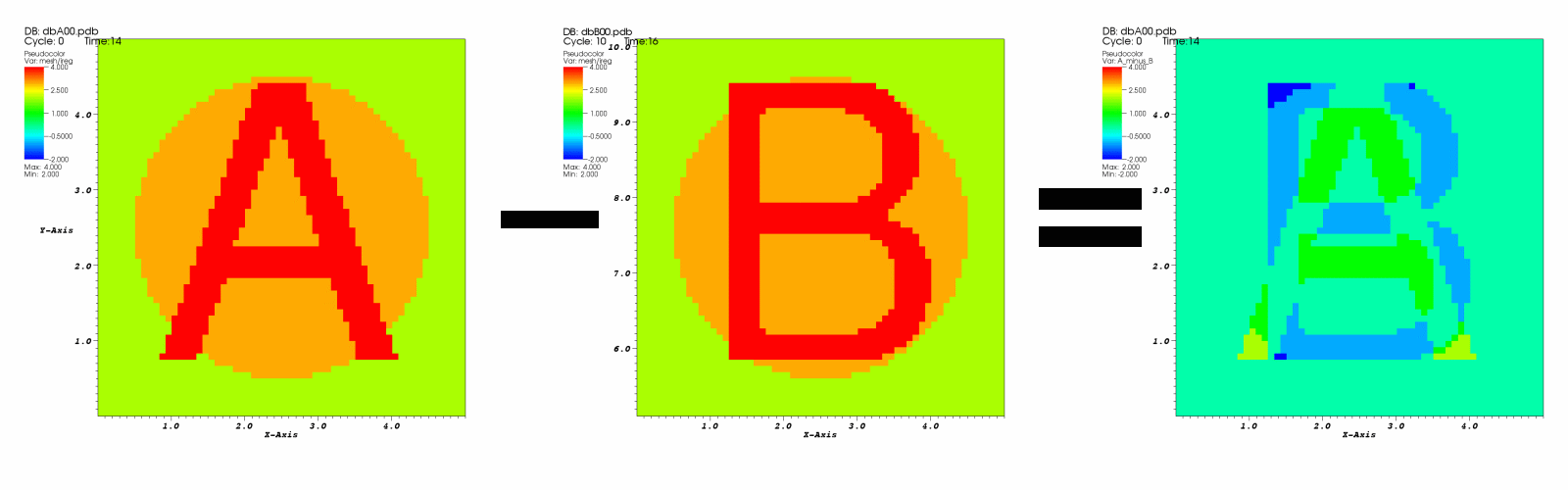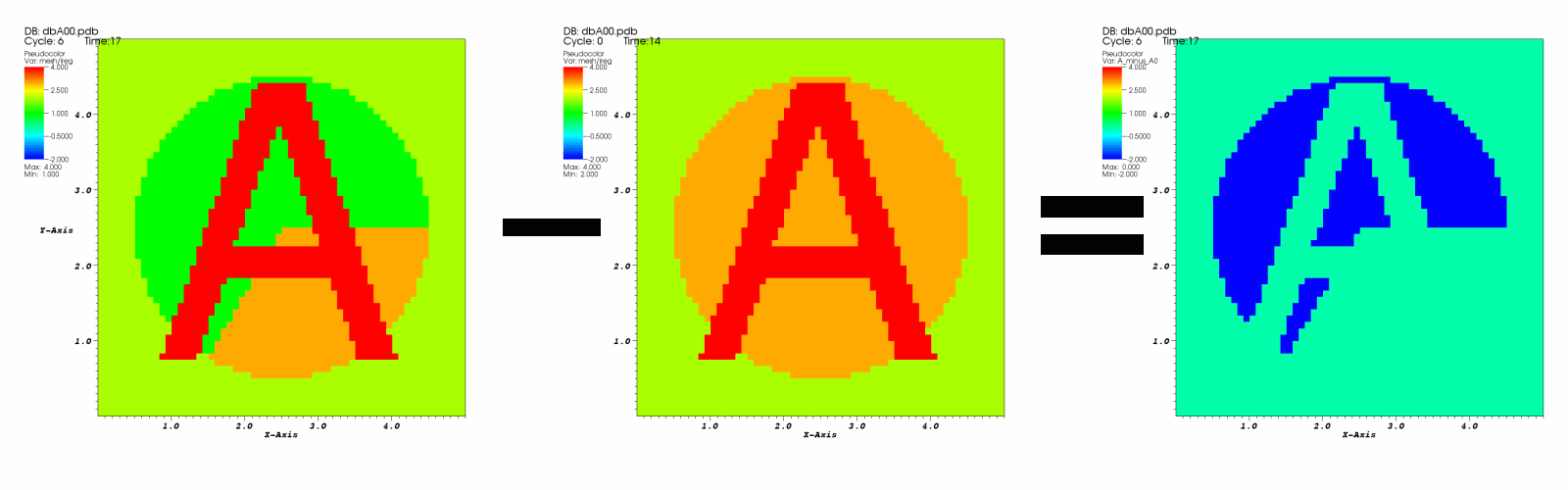12.3. Database comparison¶

Comparing the results of multiple related simulation databases is one of VisIt’s main uses. Users can plot multiple databases in the same window or adjacent windows, allowing comparison of plots visually. In addition to these visual modes of comparison, VisIt also supports more direct numerical comparison through the expression system. Database comparison allows users to plot direct differences between two databases or between different time states in the same database including even in the definition of time derivatives.

Numerical database comparisons use special expressions called Cross-Mesh Field Evaluation (CMFE) expressions, pos_cmfe() and conn_cmfe(), which are capable of mapping a field from one mesh, the donor, onto another mesh, the target. The name conn_cmfe() stands for connectivity-based cross mesh field evaluation (CMFE). It is a specialization of position-based cmfe, pos_cmfe(), for cases in which donor and target meshes be topologically congruent (e.g. size, connectivity, decomposition, etc. are identical). More information on CMFE expressions are found in the Cross-Mesh Field Evaluation (CMFE) section of the Exprssions chapter. There is also a helpful wizard, the Data Level Comparison Wizard, that simplifies the process of defining comparison expressions. Here, we walk through a few basic examples of using CMFE expressions and demonstrate how to use them in comparisons.

12.3.1. Plotting the difference between two databases¶

The typical case is where two slightly different databases time series have been generated from the same simulation code and the user wishes to work with the difference between the two databases and to have this difference update as the time slider is changed.

<mesh/ireg> - conn_cmfe(</usr/local/visit/data/dbB00.pdbid:mesh/ireg>, <mesh>)

In the above expression, the first argument to conn_cmfe() serves as the donor field and the second argument is the target mesh. This expression is a simple difference operation of database A minus database B. Note the special id time specification syntax before the colon but after the file system path in the first argument conn_cmfe(). The i means to interpret the number in brackets,  as a time state index. The d means to further interpret that number as an index difference from the current time slider index. This syntax is described in greater detail in the section describing pos_cmfe().

The assumption made by this expression is that database A is the active database and the user wishes to map database B onto it to subtract it from database A’s mesh/ireg variable. In this example, database B’s mesh/ireg field is being mapped onto database A’s mesh and their difference is then being taken. Figure 12.15 illustrates the database differencing operation.Fig. 12.15 Database B subtracted from database A

12.3.2. Plotting values relative to the first time state¶

Plotting a variable relative to its initial values can be important for understanding how the variable has changed over time. The conn_cmfe expression is also used to plot values from one time state relative to the values at the current time state. Consider the following expression:

<mesh/ireg> - conn_cmfe(</usr/local/visit/data/dbA00.pdbi:mesh/ireg>, mesh)

The above expression subtracts the value of mesh/ireg at time state zero (in the i without the d means to always map absolute time index zero from the donor) from the value of mesh/ireg at the current time As the time slider is changed, the values for the active database will change but the part of the expression using conn_cmfe, which in this case uses the first database time state, will not change. This allows users to create expressions that compare the current time state to a fixed time state.Fig. 12.16 Time state 6 minus time state 0

12.3.3. Plotting time derivatives¶

Plotting time derivatives is much like plotting the difference between the current time state and a fixed time state except that instead of being fixed, the second time state being compared is free to move relative to the current time state. To plot a simple time derivative such as the current time state minus the last time state, create an expression similar to the following expression:

<mesh/ireg> - conn_cmfe(</usr/local/visit/data/dbA00.pdb[-1]id:mesh/ireg>, mesh)

The important piece of the above expression is its use of “[-1]id” to specify a time state delta of -1, which means add -1 to the current time state to get the time state whose data will be used in the conn_cmfe calculation. You could provide different values for the time state in the [] operator. Substituting a value of 3, for example, would make the conn_cmfe expression consider the data for 3 time states beyond the current time state. If you use a time state delta, which always uses the “d” suffix, the time state being considered is always relative to the current time state. This means that as you change time states for the active database using the time slider, the plots that use the conn_cmfe expression will update properly. Figure 12.17 shows an example plot of a time derivative.Fig. 12.17 Plot of a variable and its time derivative plot